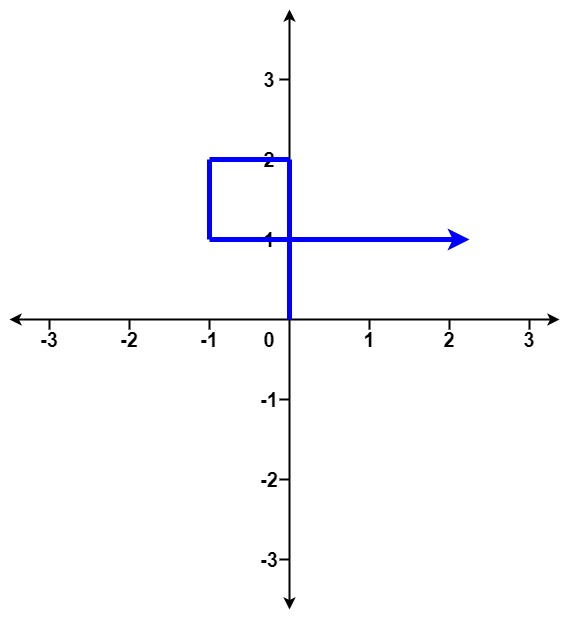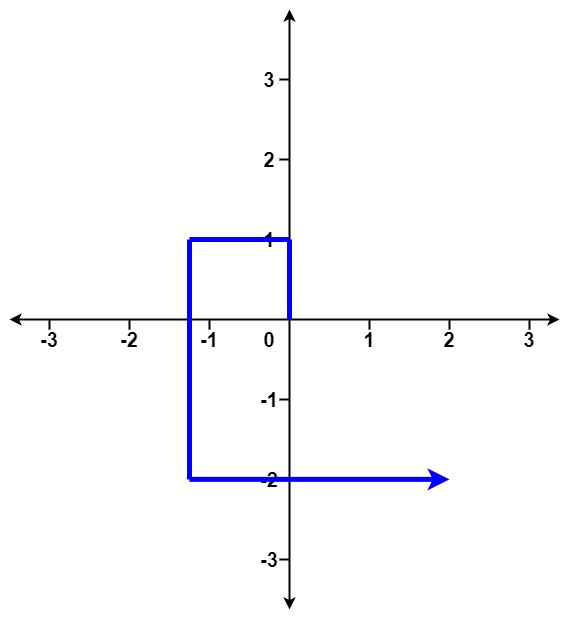304 North Cardinal St.
Dorchester Center, MA 02124

# Self Crossing LeetCode Solution

## Problem – Self Crossing LeetCode Solution

You are given an array of integers `distance`.

You start at point `(0,0)` on an X-Y plane and you move `distance` meters to the north, then `distance` meters to the west, `distance` meters to the south, `distance` meters to the east, and so on. In other words, after each move, your direction changes counter-clockwise.

Return `true` if your path crosses itself, and `false` if it does not.

Example 1:``````Input: distance = [2,1,1,2]
Output: true
``````

Example 2:``````Input: distance = [1,2,3,4]
Output: false
``````

Example 3:``````Input: distance = [1,1,1,1]
Output: true
``````

Constraints:

• `1 <= distance.length <= 105`
• `1 <= distance[i] <= 105`

## Self Crossing LeetCode Solution in Java

``````// Categorize the self-crossing scenarios, there are 3 of them:
// 1. Fourth line crosses first line and works for fifth line crosses second line and so on...
// 2. Fifth line meets first line and works for the lines after
// 3. Sixth line crosses first line and works for the lines after
public class Solution {
public boolean isSelfCrossing(int[] x) {
int l = x.length;
if(l <= 3) return false;

for(int i = 3; i < l; i++){
if(x[i] >= x[i-2] && x[i-1] <= x[i-3]) return true;  //Fourth line crosses first line and onward
if(i >=4)
{
if(x[i-1] == x[i-3] && x[i] + x[i-4] >= x[i-2]) return true; // Fifth line meets first line and onward
}
if(i >=5)
{
if(x[i-2] - x[i-4] >= 0 && x[i] >= x[i-2] - x[i-4] && x[i-1] >= x[i-3] - x[i-5] && x[i-1] <= x[i-3]) return true;  // Sixth line crosses first line and onward
}
}
return false;
}
}
``````

## Self Crossing LeetCode Solution in Python

``````def isSelfCrossing(self, x):
return any(d >= b > 0 and (a >= c or a >= c-e >= 0 and f >= d-b)
for a, b, c, d, e, f in ((x[i:i+6] +  * 6)[:6]
for i in xrange(len(x))))
``````

## Self Crossing LeetCode Solution in C++

``````class Solution
{
public:
bool isSelfCrossing(vector<int>& x)
{
x.insert(x.begin(), 4, 0);

int len = x.size();
int i = 4;

// outer spiral
for (; i < len && x[i] > x[i - 2]; i++);

if (i == len) return false;

// check border
if (x[i] >= x[i - 2] - x[i - 4])
{
x[i - 1] -= x[i - 3];
}

// inner spiral
for (i++; i < len && x[i] < x[i - 2]; i++);

return i != len;
}
};
``````
##### Self Crossing LeetCode Solution Review:

In our experience, we suggest you solve this Self Crossing LeetCode Solution and gain some new skills from Professionals completely free and we assure you will be worth it.

If you are stuck anywhere between any coding problem, just visit Queslers to get the Self Crossing LeetCode Solution

Find on Leetcode

##### Conclusion:

I hope this Self Crossing LeetCode Solution would be useful for you to learn something new from this problem. If it helped you then don’t forget to bookmark our site for more Coding Solutions.

This Problem is intended for audiences of all experiences who are interested in learning about Data Science in a business context; there are no prerequisites.

Keep Learning!

More Coding Solutions >>

LeetCode Solutions

Hacker Rank Solutions

CodeChef Solutions